# Classification Basic Concepts Decision Trees and Model Evaluation

• Slides: 67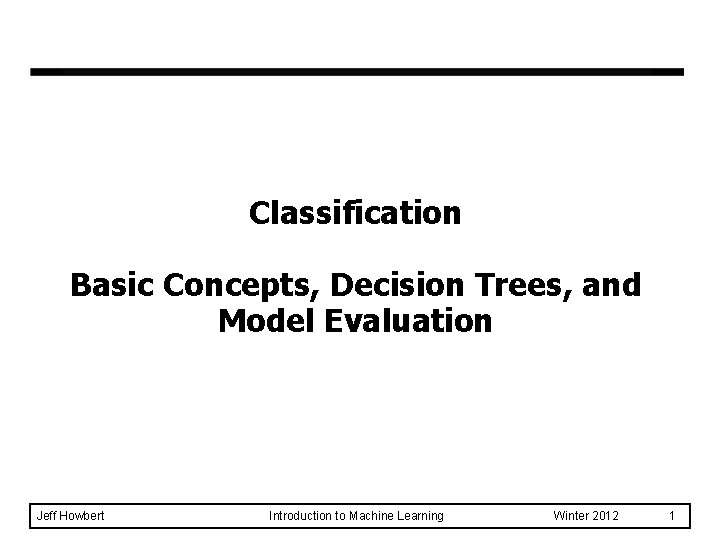Classification Basic Concepts, Decision Trees, and Model Evaluation Jeff Howbert Introduction to Machine Learning Winter 2012 1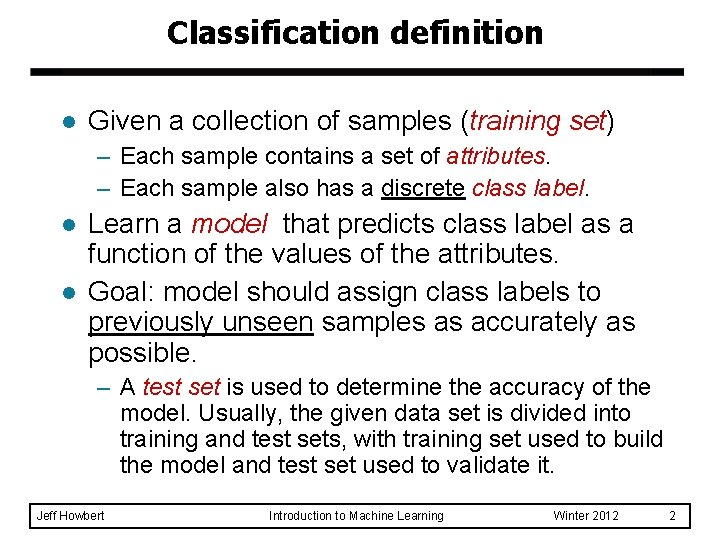Classification definition l Given a collection of samples (training set) – Each sample contains a set of attributes. – Each sample also has a discrete class label. l l Learn a model that predicts class label as a function of the values of the attributes. Goal: model should assign class labels to previously unseen samples as accurately as possible. – A test set is used to determine the accuracy of the model. Usually, the given data set is divided into training and test sets, with training set used to build the model and test set used to validate it. Jeff Howbert Introduction to Machine Learning Winter 2012 2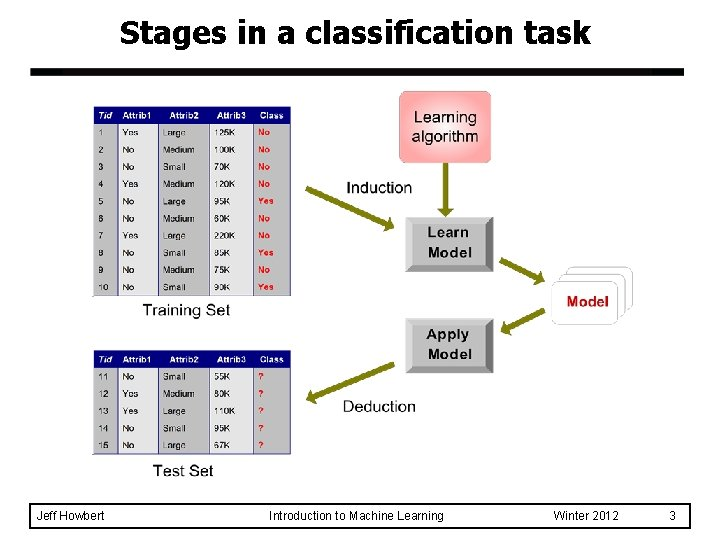Stages in a classification task Jeff Howbert Introduction to Machine Learning Winter 2012 3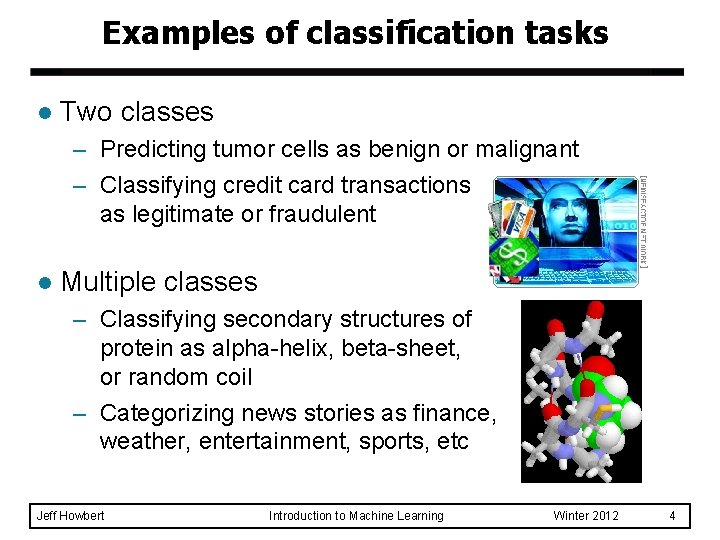Examples of classification tasks l Two classes – Predicting tumor cells as benign or malignant – Classifying credit card transactions as legitimate or fraudulent l Multiple classes – Classifying secondary structures of protein as alpha-helix, beta-sheet, or random coil – Categorizing news stories as finance, weather, entertainment, sports, etc Jeff Howbert Introduction to Machine Learning Winter 2012 4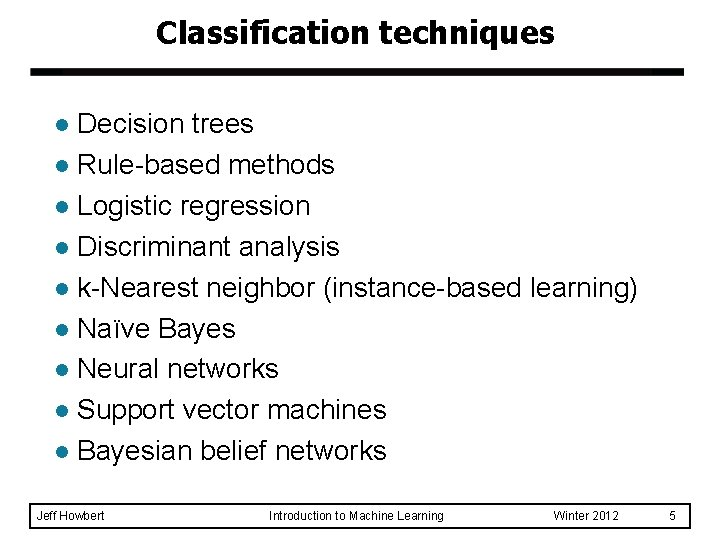Classification techniques Decision trees l Rule-based methods l Logistic regression l Discriminant analysis l k-Nearest neighbor (instance-based learning) l Naïve Bayes l Neural networks l Support vector machines l Bayesian belief networks l Jeff Howbert Introduction to Machine Learning Winter 2012 5Example of a decision tree in m no al in n al om r io at s as l c splitting nodes Refund Yes No NO Mar. St Single, Divorced Married Tax. Inc < 80 K NO NO > 80 K YES classification nodes training data Jeff Howbert model: decision tree Introduction to Machine Learning Winter 2012 6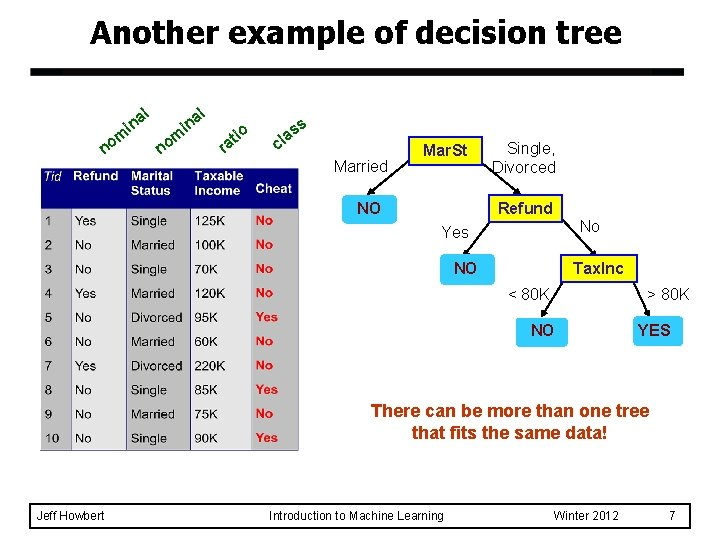Another example of decision tree a in m no l al n i n om r io at ss a cl Married Mar. St NO Single, Divorced Refund No Yes NO Tax. Inc < 80 K > 80 K NO YES There can be more than one tree that fits the same data! Jeff Howbert Introduction to Machine Learning Winter 2012 7Decision tree classification task Decision Tree Jeff Howbert Introduction to Machine Learning Winter 2012 8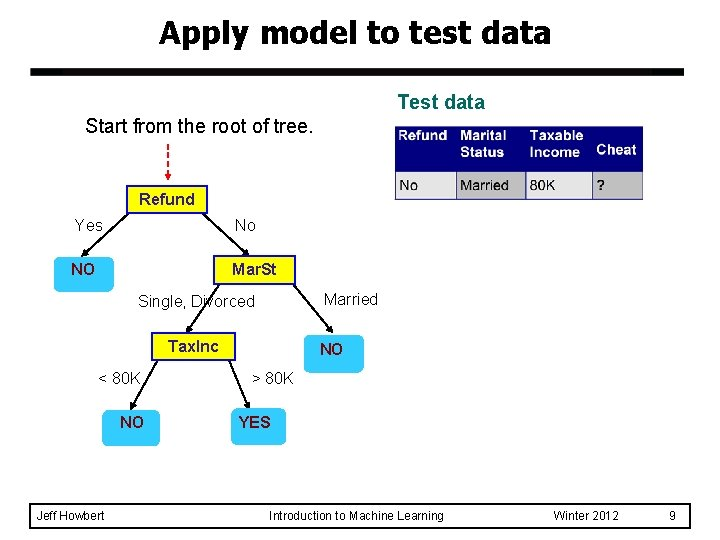Apply model to test data Test data Start from the root of tree. Refund Yes No NO Mar. St Married Single, Divorced Tax. Inc < 80 K NO Jeff Howbert NO > 80 K YES Introduction to Machine Learning Winter 2012 9Apply model to test data Test data Refund Yes No NO Mar. St Married Single, Divorced Tax. Inc < 80 K NO Jeff Howbert NO > 80 K YES Introduction to Machine Learning Winter 2012 10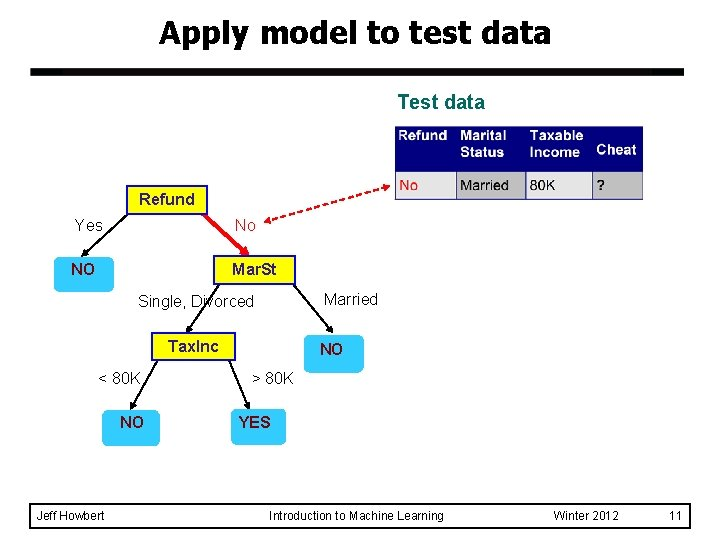Apply model to test data Test data Refund Yes No NO Mar. St Married Single, Divorced Tax. Inc < 80 K NO Jeff Howbert NO > 80 K YES Introduction to Machine Learning Winter 2012 11Apply model to test data Test data Refund Yes No NO Mar. St Married Single, Divorced Tax. Inc < 80 K NO Jeff Howbert NO > 80 K YES Introduction to Machine Learning Winter 2012 12Apply model to test data Test data Refund Yes No NO Mar. St Married Single, Divorced Tax. Inc < 80 K NO Jeff Howbert NO > 80 K YES Introduction to Machine Learning Winter 2012 13Apply model to test data Test data Refund Yes No NO Mar. St Married Single, Divorced Tax. Inc < 80 K NO Jeff Howbert Assign Cheat to “No” NO > 80 K YES Introduction to Machine Learning Winter 2012 14Decision tree classification task Decision Tree Jeff Howbert Introduction to Machine Learning Winter 2012 15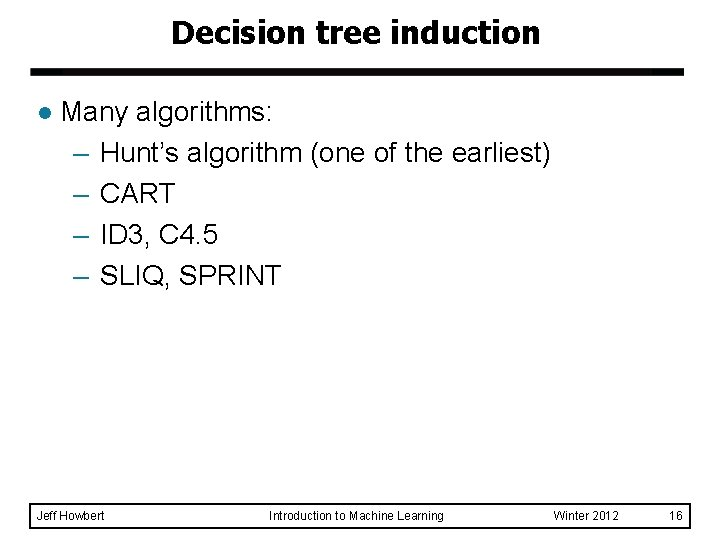Decision tree induction l Many algorithms: – Hunt’s algorithm (one of the earliest) – CART – ID 3, C 4. 5 – SLIQ, SPRINT Jeff Howbert Introduction to Machine Learning Winter 2012 16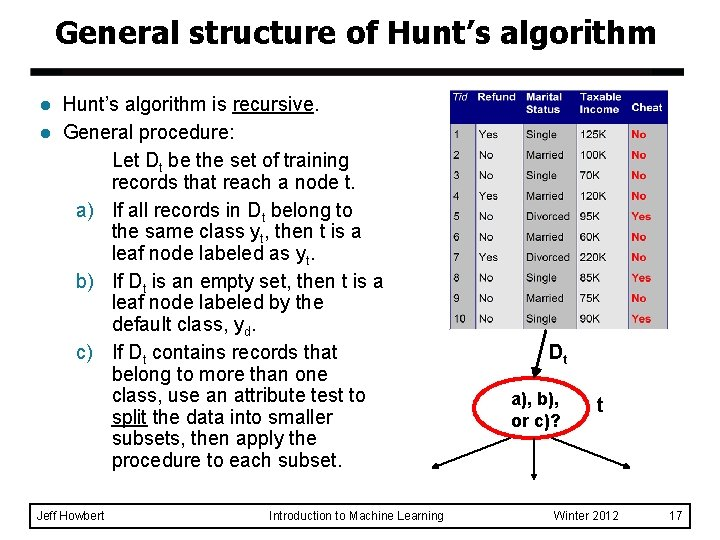General structure of Hunt’s algorithm l l Hunt’s algorithm is recursive. General procedure: Let Dt be the set of training records that reach a node t. a) If all records in Dt belong to the same class yt, then t is a leaf node labeled as yt. b) If Dt is an empty set, then t is a leaf node labeled by the default class, yd. c) If Dt contains records that belong to more than one class, use an attribute test to split the data into smaller subsets, then apply the procedure to each subset. Jeff Howbert Introduction to Machine Learning Dt a), b), or c)? t Winter 2012 17Applying Hunt’s algorithm Don’t Cheat Refund Yes No Yes Don’t Cheat No Don’t Cheat Marital Status Single, Divorced Refund Yes Don’t Cheat Single, Divorced Cheat No Don’t Cheat Marital Status Married Don’t Cheat Taxable Income Jeff Howbert Married < 80 K >= 80 K Don’t Cheat Introduction to Machine Learning Winter 2012 18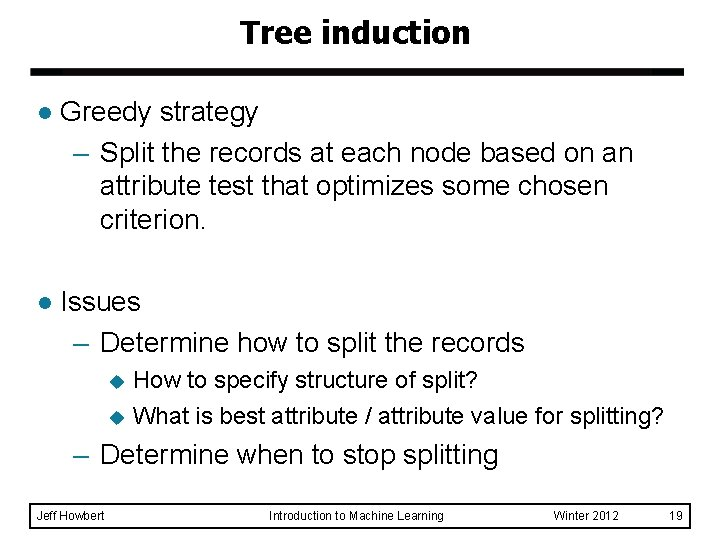Tree induction l Greedy strategy – Split the records at each node based on an attribute test that optimizes some chosen criterion. l Issues – Determine how to split the records How to specify structure of split? u What is best attribute / attribute value for splitting? u – Determine when to stop splitting Jeff Howbert Introduction to Machine Learning Winter 2012 19Tree induction l Greedy strategy – Split the records at each node based on an attribute test that optimizes some chosen criterion. l Issues – Determine how to split the records How to specify structure of split? u What is best attribute / attribute value for splitting? u – Determine when to stop splitting Jeff Howbert Introduction to Machine Learning Winter 2012 20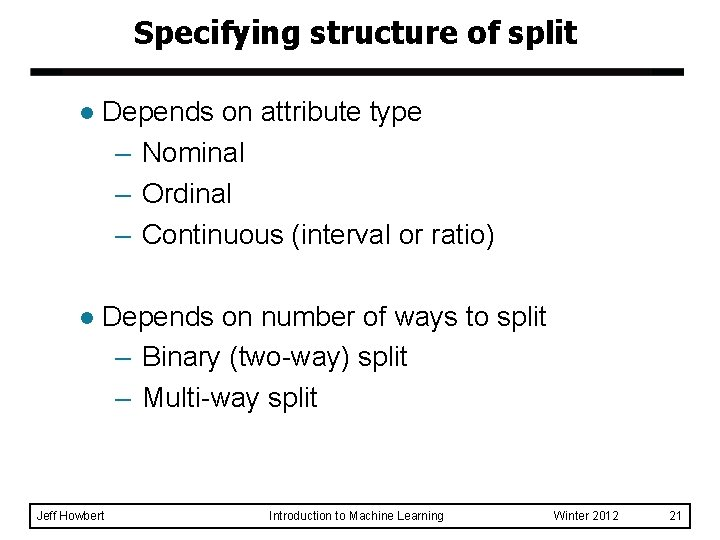Specifying structure of split l Depends on attribute type – Nominal – Ordinal – Continuous (interval or ratio) l Depends on number of ways to split – Binary (two-way) split – Multi-way split Jeff Howbert Introduction to Machine Learning Winter 2012 21Splitting based on nominal attributes l Multi-way split: Use as many partitions as distinct values. Car. Type Family Luxury Sports l Binary split: Divides values into two subsets. Need to find optimal partitioning. {Sports, Luxury} Jeff Howbert Car. Type {Family} OR Introduction to Machine Learning {Family, Luxury} Car. Type {Sports} Winter 2012 22Splitting based on ordinal attributes l Multi-way split: Use as many partitions as distinct values. Size Small Medium l Binary split: Divides values into two subsets. Need to find optimal partitioning. {Small, Medium} l Large Size {Large} What about this split? Jeff Howbert OR {Small, Large} Introduction to Machine Learning {Medium, Large} Size {Small} Size {Medium} Winter 2012 23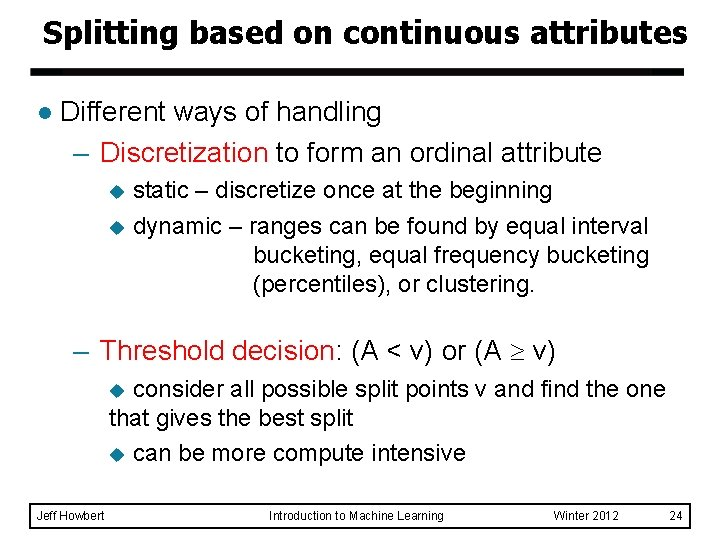Splitting based on continuous attributes l Different ways of handling – Discretization to form an ordinal attribute static – discretize once at the beginning u dynamic – ranges can be found by equal interval bucketing, equal frequency bucketing (percentiles), or clustering. u – Threshold decision: (A < v) or (A v) consider all possible split points v and find the one that gives the best split u can be more compute intensive u Jeff Howbert Introduction to Machine Learning Winter 2012 24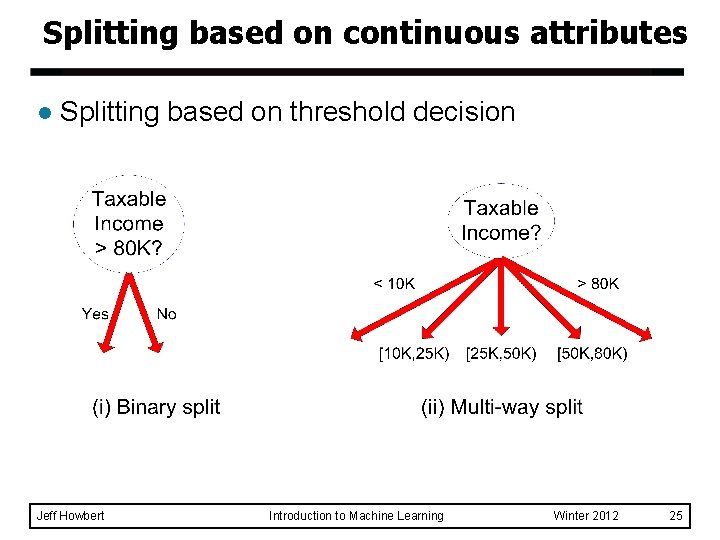Splitting based on continuous attributes l Splitting based on threshold decision Jeff Howbert Introduction to Machine Learning Winter 2012 25Tree induction l Greedy strategy – Split the records at each node based on an attribute test that optimizes some chosen criterion. l Issues – Determine how to split the records How to specify structure of split? u What is best attribute / attribute value for splitting? u – Determine when to stop splitting Jeff Howbert Introduction to Machine Learning Winter 2012 26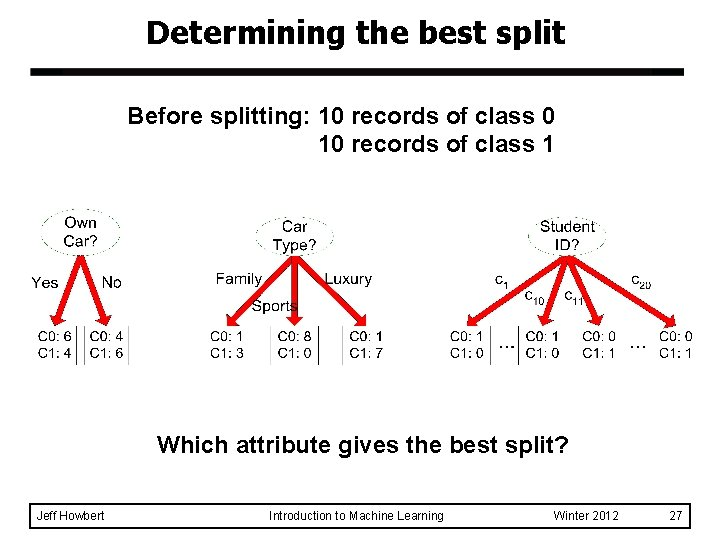Determining the best split Before splitting: 10 records of class 0 10 records of class 1 Which attribute gives the best split? Jeff Howbert Introduction to Machine Learning Winter 2012 27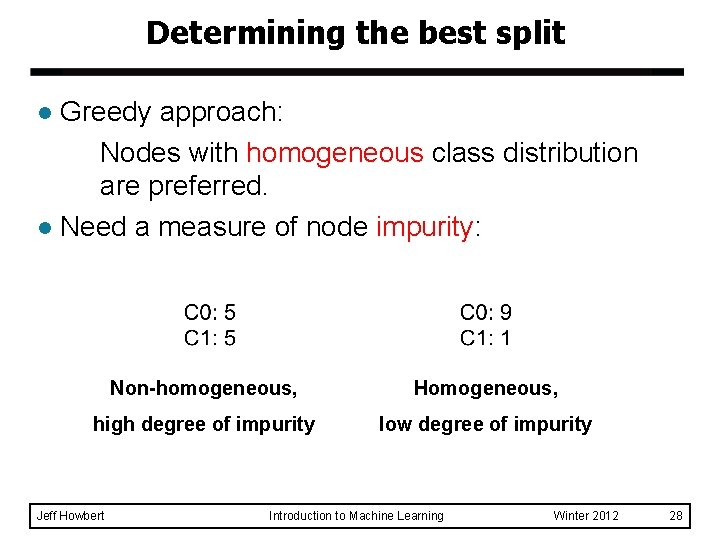Determining the best split Greedy approach: Nodes with homogeneous class distribution are preferred. l Need a measure of node impurity: l Non-homogeneous, Homogeneous, high degree of impurity low degree of impurity Jeff Howbert Introduction to Machine Learning Winter 2012 28Measures of node impurity Jeff Howbert l Gini index l Entropy l Misclassification error Introduction to Machine Learning Winter 2012 29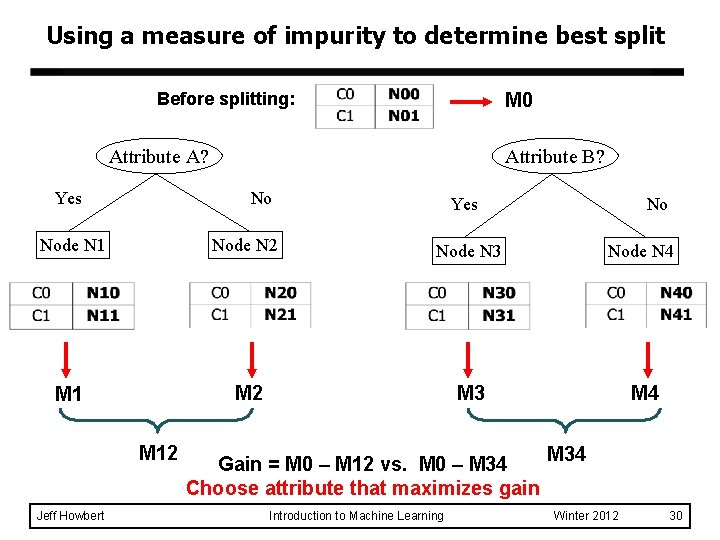Using a measure of impurity to determine best split Before splitting: M 0 Attribute A? Yes Attribute B? No Yes No Node N 1 Node N 2 Node N 3 Node N 4 M 1 M 2 M 3 M 4 M 12 Jeff Howbert Gain = M 0 – M 12 vs. M 0 – M 34 Choose attribute that maximizes gain Introduction to Machine Learning M 34 Winter 2012 30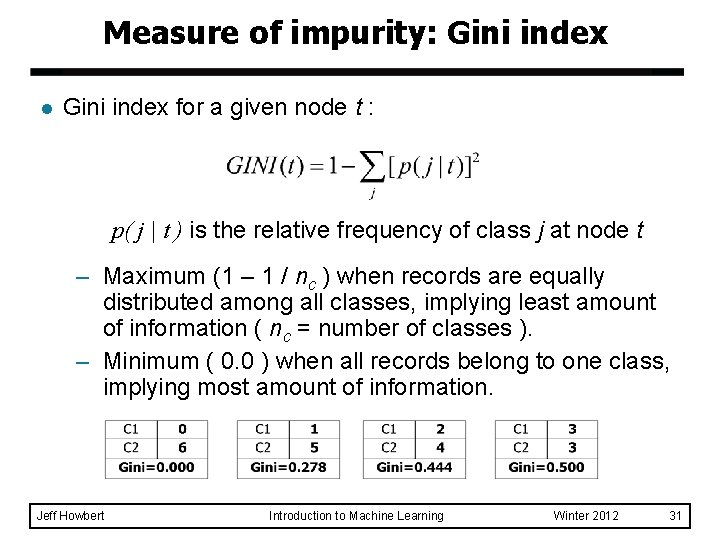Measure of impurity: Gini index l Gini index for a given node t : p( j | t ) is the relative frequency of class j at node t – Maximum (1 – 1 / nc ) when records are equally distributed among all classes, implying least amount of information ( nc = number of classes ). – Minimum ( 0. 0 ) when all records belong to one class, implying most amount of information. Jeff Howbert Introduction to Machine Learning Winter 2012 31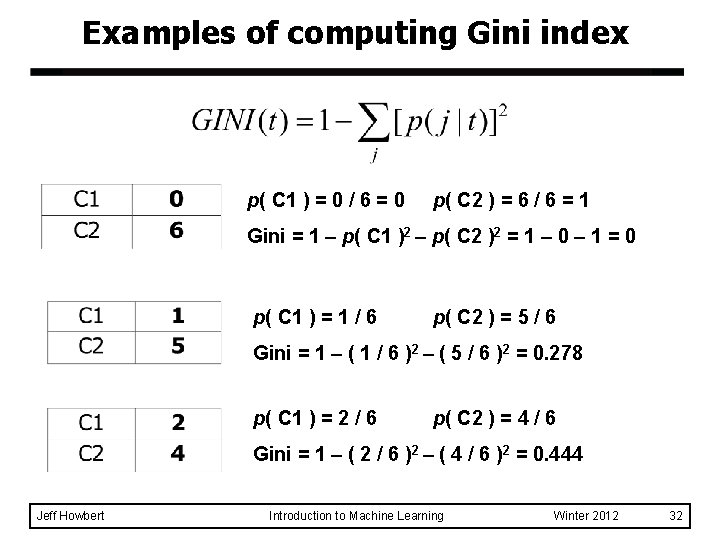Examples of computing Gini index p( C 1 ) = 0 / 6 = 0 p( C 2 ) = 6 / 6 = 1 Gini = 1 – p( C 1 )2 – p( C 2 )2 = 1 – 0 – 1 = 0 p( C 1 ) = 1 / 6 p( C 2 ) = 5 / 6 Gini = 1 – ( 1 / 6 )2 – ( 5 / 6 )2 = 0. 278 p( C 1 ) = 2 / 6 p( C 2 ) = 4 / 6 Gini = 1 – ( 2 / 6 )2 – ( 4 / 6 )2 = 0. 444 Jeff Howbert Introduction to Machine Learning Winter 2012 32Splitting based on Gini index l l Used in CART, SLIQ, SPRINT. When a node t is split into k partitions (child nodes), the quality of split is computed as, where Jeff Howbert ni = number of records at child node i n = number of records at parent node t Introduction to Machine Learning Winter 2012 33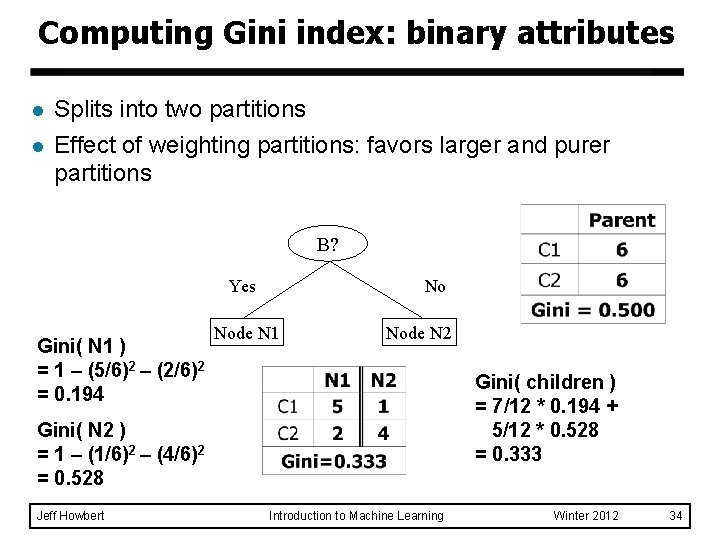Computing Gini index: binary attributes l l Splits into two partitions Effect of weighting partitions: favors larger and purer partitions B? Yes Gini( N 1 ) = 1 – (5/6)2 – (2/6)2 = 0. 194 No Node N 1 Node N 2 Gini( children ) = 7/12 * 0. 194 + 5/12 * 0. 528 = 0. 333 Gini( N 2 ) = 1 – (1/6)2 – (4/6)2 = 0. 528 Jeff Howbert Introduction to Machine Learning Winter 2012 34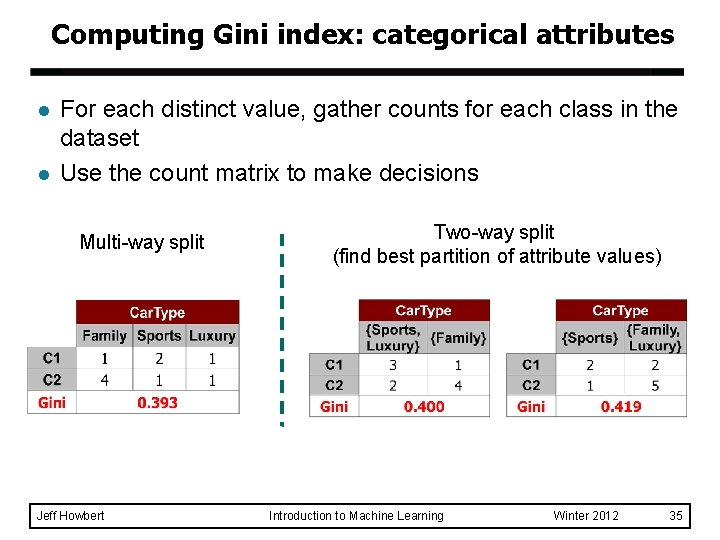Computing Gini index: categorical attributes l l For each distinct value, gather counts for each class in the dataset Use the count matrix to make decisions Multi-way split Jeff Howbert Two-way split (find best partition of attribute values) Introduction to Machine Learning Winter 2012 35Computing Gini index: continuous attributes l l Make binary split based on a threshold (splitting) value of attribute Number of possible splitting values = (number of distinct values attribute has at that node) - 1 Each splitting value v has a count matrix associated with it – Class counts in each of the partitions, A < v and A v Simple method to choose best v – For each v, scan the attribute values at the node to gather count matrix, then compute its Gini index. – Computationally inefficient! Repetition of work. Jeff Howbert Introduction to Machine Learning Winter 2012 36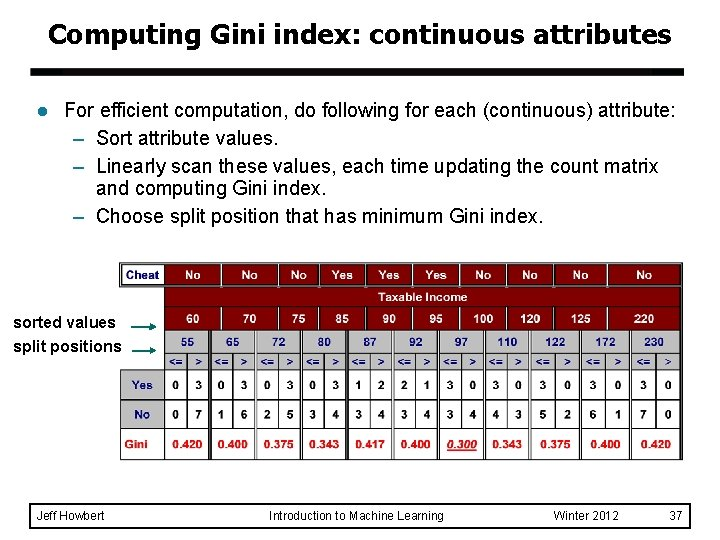Computing Gini index: continuous attributes l For efficient computation, do following for each (continuous) attribute: – Sort attribute values. – Linearly scan these values, each time updating the count matrix and computing Gini index. – Choose split position that has minimum Gini index. sorted values split positions Jeff Howbert Introduction to Machine Learning Winter 2012 37Comparison among splitting criteria For a two-class problem: Jeff Howbert Introduction to Machine Learning Winter 2012 38Tree induction l Greedy strategy – Split the records at each node based on an attribute test that optimizes some chosen criterion. l Issues – Determine how to split the records How to specify structure of split? u What is best attribute / attribute value for splitting? u – Determine when to stop splitting Jeff Howbert Introduction to Machine Learning Winter 2012 39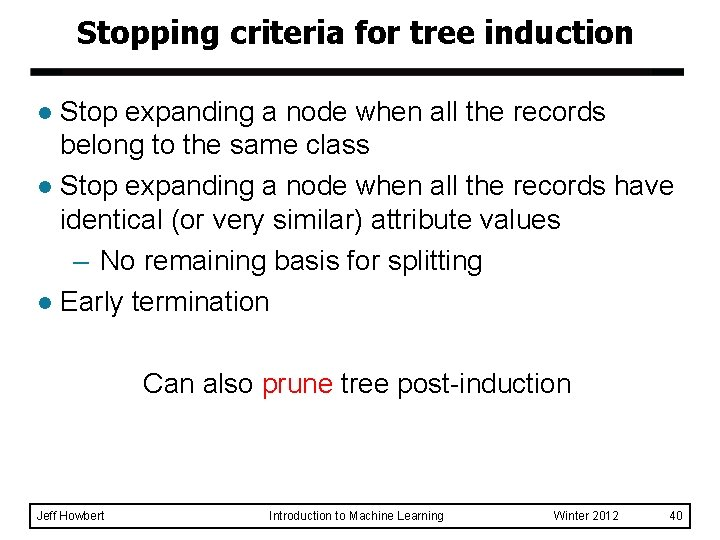Stopping criteria for tree induction Stop expanding a node when all the records belong to the same class l Stop expanding a node when all the records have identical (or very similar) attribute values – No remaining basis for splitting l Early termination l Can also prune tree post-induction Jeff Howbert Introduction to Machine Learning Winter 2012 40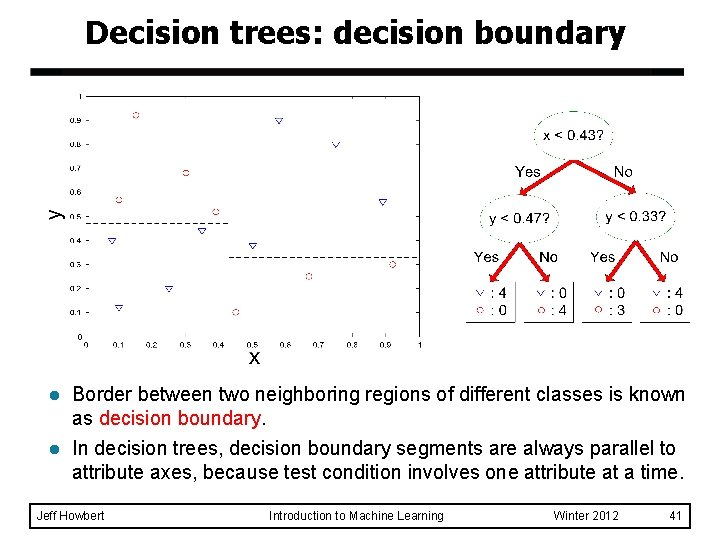Decision trees: decision boundary l Border between two neighboring regions of different classes is known as decision boundary. l In decision trees, decision boundary segments are always parallel to attribute axes, because test condition involves one attribute at a time. Jeff Howbert Introduction to Machine Learning Winter 2012 41Classification with decision trees Advantages: – Inexpensive to construct – Extremely fast at classifying unknown records – Easy to interpret for small-sized trees – Accuracy comparable to other classification techniques for many simple data sets l Disadvantages: – Easy to overfit – Decision boundary restricted to being parallel to attribute axes l Jeff Howbert Introduction to Machine Learning Winter 2012 42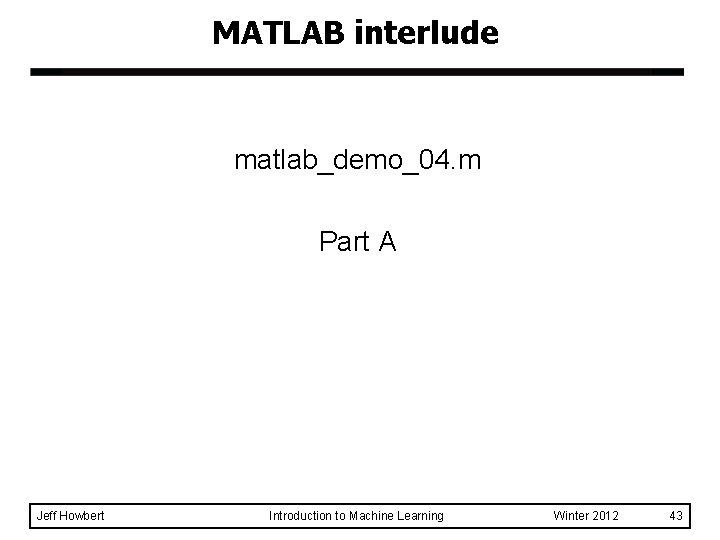MATLAB interlude matlab_demo_04. m Part A Jeff Howbert Introduction to Machine Learning Winter 2012 43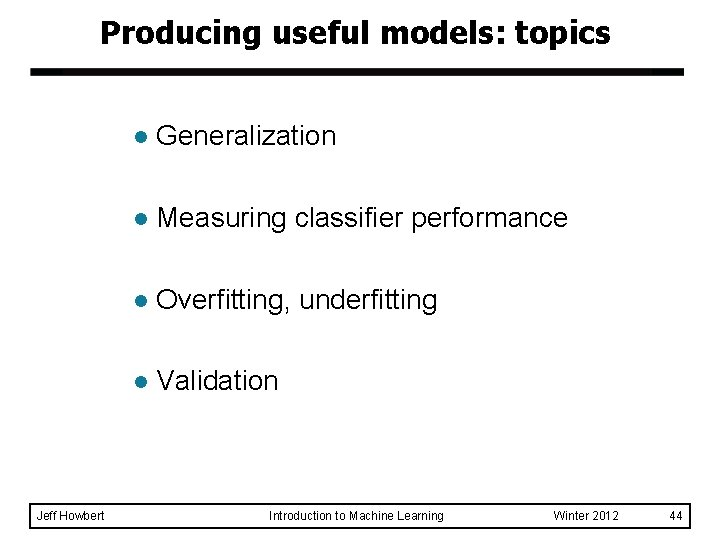Producing useful models: topics Jeff Howbert l Generalization l Measuring classifier performance l Overfitting, underfitting l Validation Introduction to Machine Learning Winter 2012 44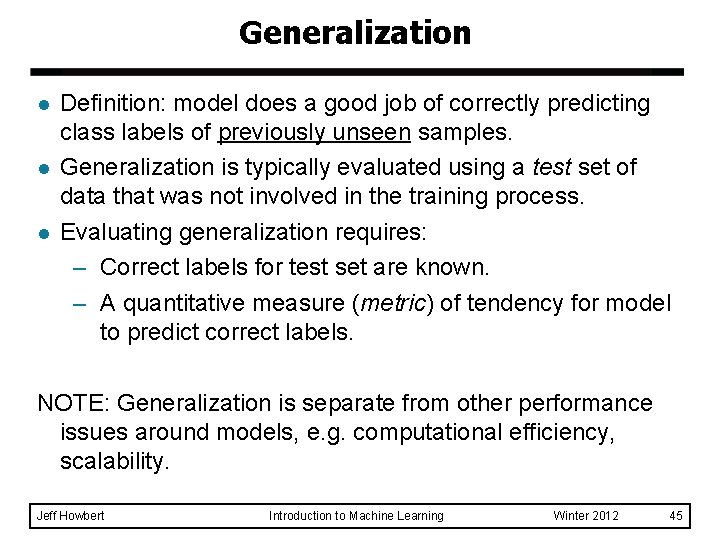Generalization l l l Definition: model does a good job of correctly predicting class labels of previously unseen samples. Generalization is typically evaluated using a test set of data that was not involved in the training process. Evaluating generalization requires: – Correct labels for test set are known. – A quantitative measure (metric) of tendency for model to predict correct labels. NOTE: Generalization is separate from other performance issues around models, e. g. computational efficiency, scalability. Jeff Howbert Introduction to Machine Learning Winter 2012 45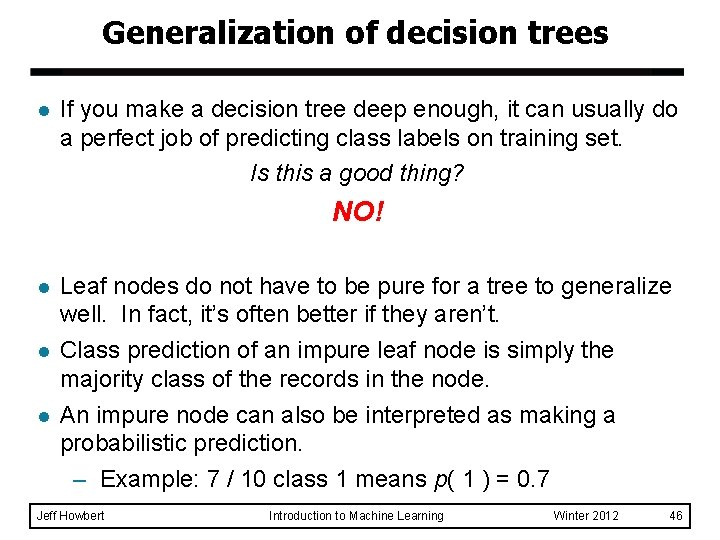Generalization of decision trees l If you make a decision tree deep enough, it can usually do a perfect job of predicting class labels on training set. Is this a good thing? NO! l l l Leaf nodes do not have to be pure for a tree to generalize well. In fact, it’s often better if they aren’t. Class prediction of an impure leaf node is simply the majority class of the records in the node. An impure node can also be interpreted as making a probabilistic prediction. – Example: 7 / 10 class 1 means p( 1 ) = 0. 7 Jeff Howbert Introduction to Machine Learning Winter 2012 46Metrics for classifier performance l Accuracy a = number of test samples with label correctly predicted b = number of test samples with label incorrectly predicted example u 75 samples in test set u correct class label predicted for 62 samples u wrong class label predicted for 13 samples u accuracy = 62 / 75 = 0. 827 Jeff Howbert Introduction to Machine Learning Winter 2012 47Metrics for classifier performance l Limitations of accuracy – Consider a two-class problem u number of class 1 test samples = 9990 u number of class 2 test samples = 10 – What if model predicts everything to be class 1? u accuracy is extremely high: 9990 / 10000 = 99. 9 % u but model will never correctly predict any sample in class 2 in this case accuracy is misleading and does not give a good picture of model quality u Jeff Howbert Introduction to Machine Learning Winter 2012 48Metrics for classifier performance l Confusion matrix example actual class (continued from two slides back) predicted class Jeff Howbert class 1 class 2 class 1 21 6 class 2 7 41 Introduction to Machine Learning Winter 2012 49Metrics for classifier performance l Confusion matrix – derived metrics (for two classes) class 1 predicted class (negative) class 2 (positive) Jeff Howbert actual class 1 class 2 (negative) (positive) 21 (TN) 6 (FN) 7 (FP) 41 (TP) TN: true negatives FN: false negatives FP: false positives TP: true positives Introduction to Machine Learning Winter 2012 50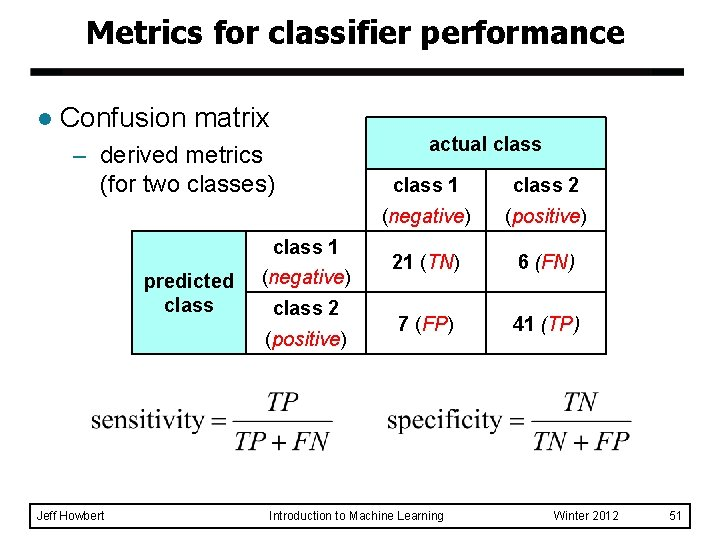Metrics for classifier performance l Confusion matrix – derived metrics (for two classes) class 1 predicted class (negative) class 2 (positive) Jeff Howbert actual class 1 class 2 (negative) (positive) 21 (TN) 6 (FN) 7 (FP) 41 (TP) Introduction to Machine Learning Winter 2012 51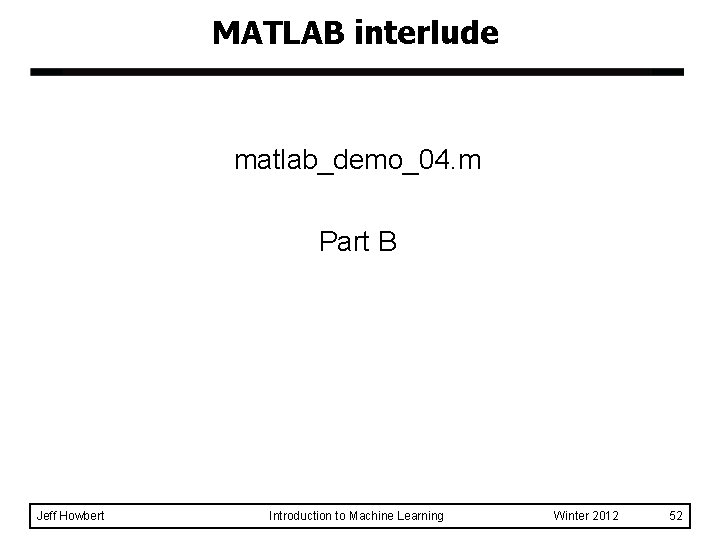MATLAB interlude matlab_demo_04. m Part B Jeff Howbert Introduction to Machine Learning Winter 2012 52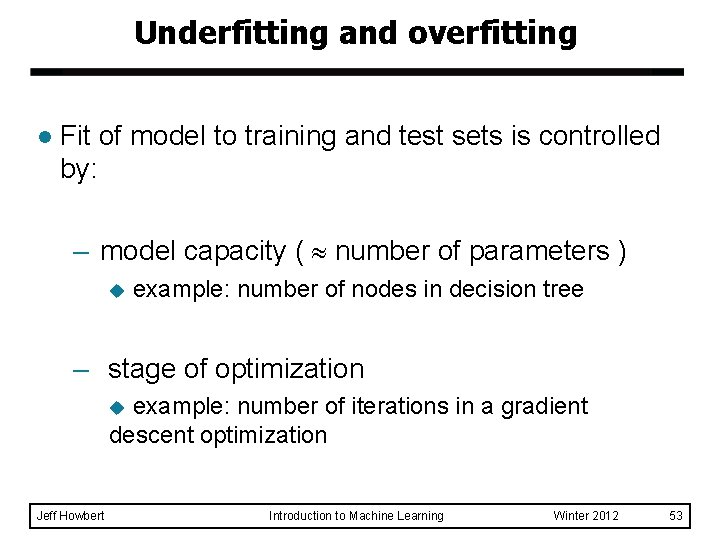Underfitting and overfitting l Fit of model to training and test sets is controlled by: – model capacity ( number of parameters ) u example: number of nodes in decision tree – stage of optimization example: number of iterations in a gradient descent optimization u Jeff Howbert Introduction to Machine Learning Winter 2012 53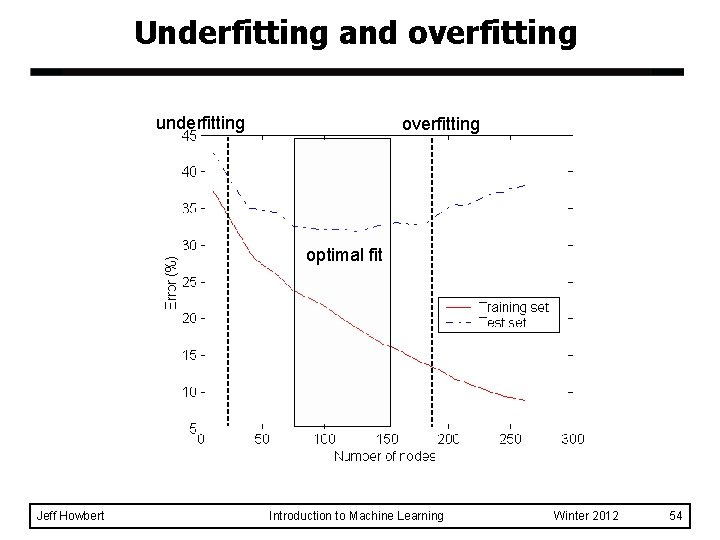Underfitting and overfitting underfitting overfitting optimal fit Jeff Howbert Introduction to Machine Learning Winter 2012 54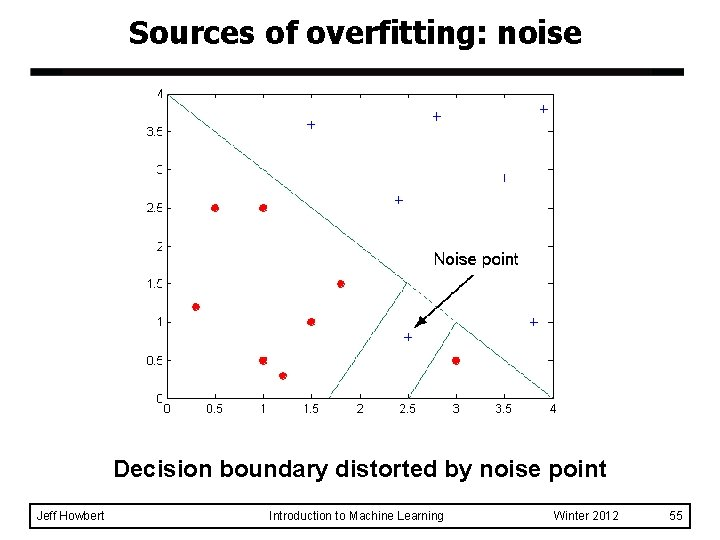Sources of overfitting: noise Decision boundary distorted by noise point Jeff Howbert Introduction to Machine Learning Winter 2012 55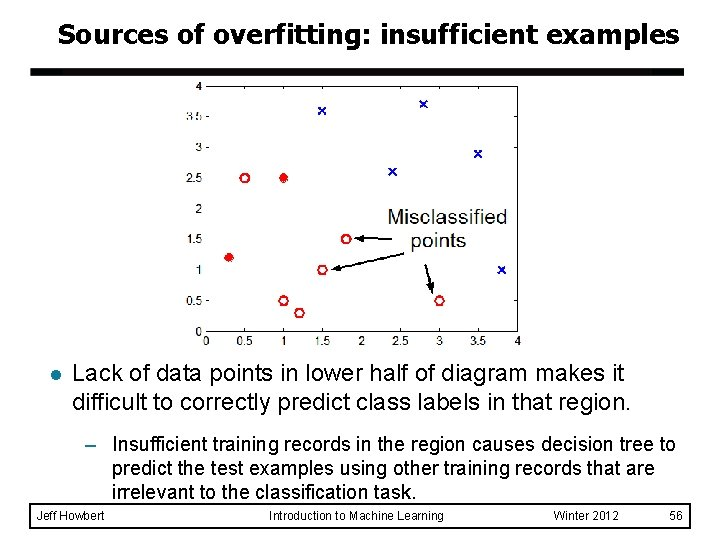Sources of overfitting: insufficient examples l Lack of data points in lower half of diagram makes it difficult to correctly predict class labels in that region. – Insufficient training records in the region causes decision tree to predict the test examples using other training records that are irrelevant to the classification task. Jeff Howbert Introduction to Machine Learning Winter 2012 56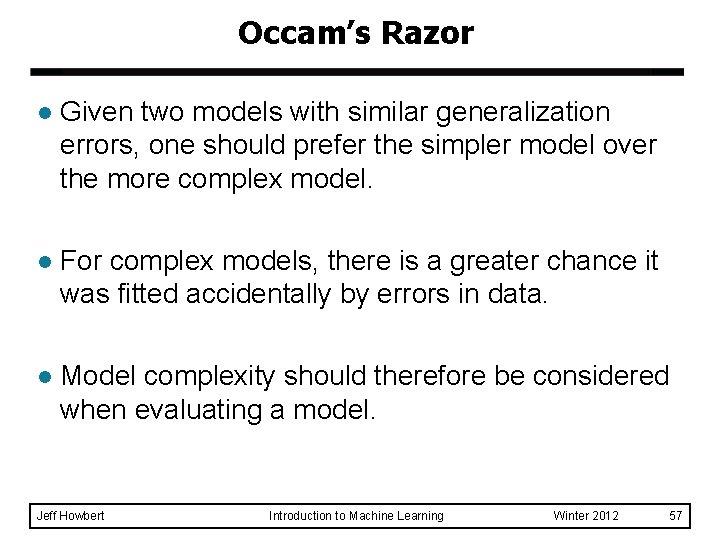Occam’s Razor l Given two models with similar generalization errors, one should prefer the simpler model over the more complex model. l For complex models, there is a greater chance it was fitted accidentally by errors in data. l Model complexity should therefore be considered when evaluating a model. Jeff Howbert Introduction to Machine Learning Winter 2012 57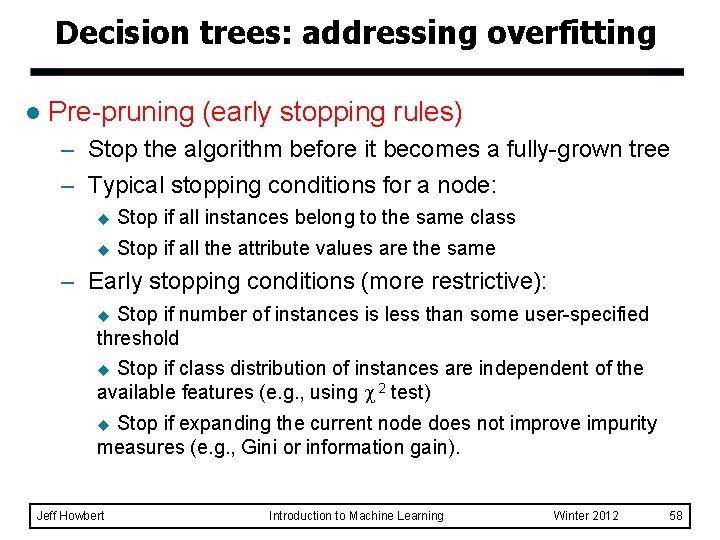Decision trees: addressing overfitting l Pre-pruning (early stopping rules) – Stop the algorithm before it becomes a fully-grown tree – Typical stopping conditions for a node: u Stop if all instances belong to the same class u Stop if all the attribute values are the same – Early stopping conditions (more restrictive): Stop if number of instances is less than some user-specified threshold u Stop if class distribution of instances are independent of the available features (e. g. , using 2 test) u Stop if expanding the current node does not improve impurity measures (e. g. , Gini or information gain). u Jeff Howbert Introduction to Machine Learning Winter 2012 58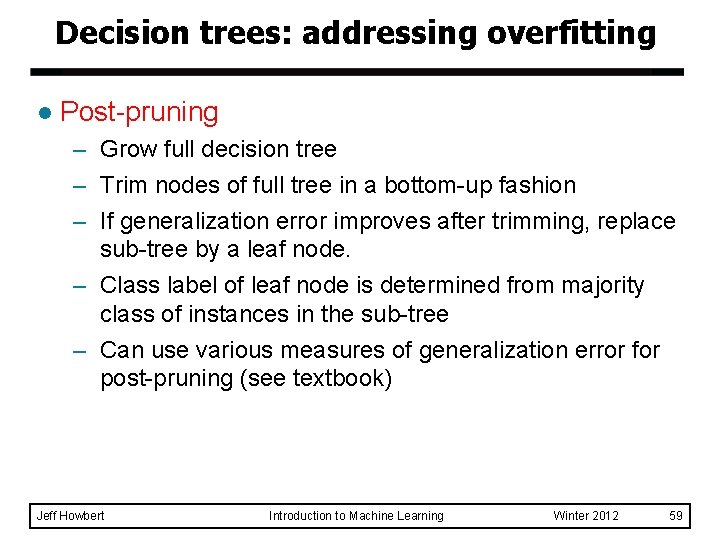Decision trees: addressing overfitting l Post-pruning – Grow full decision tree – Trim nodes of full tree in a bottom-up fashion – If generalization error improves after trimming, replace sub-tree by a leaf node. – Class label of leaf node is determined from majority class of instances in the sub-tree – Can use various measures of generalization error for post-pruning (see textbook) Jeff Howbert Introduction to Machine Learning Winter 2012 59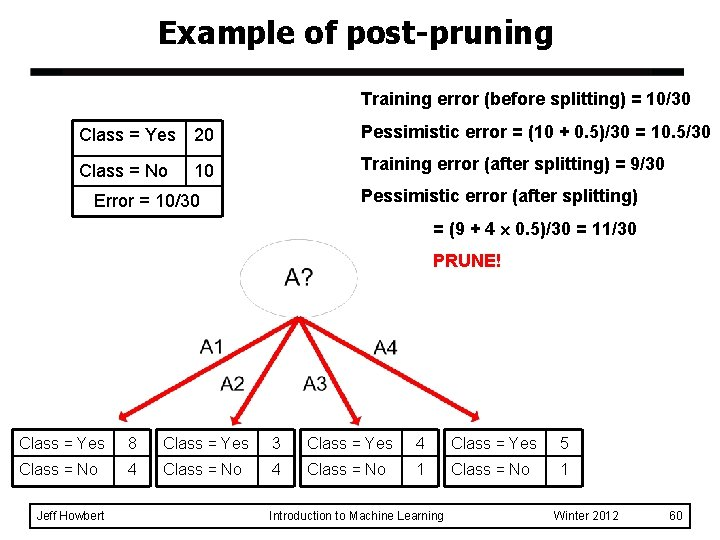Example of post-pruning Training error (before splitting) = 10/30 Class = Yes 20 Pessimistic error = (10 + 0. 5)/30 = 10. 5/30 Class = No 10 Training error (after splitting) = 9/30 Pessimistic error (after splitting) Error = 10/30 = (9 + 4 0. 5)/30 = 11/30 PRUNE! Class = Yes 8 Class = Yes 3 Class = Yes 4 Class = Yes 5 Class = No 4 Class = No 1 Jeff Howbert Introduction to Machine Learning Winter 2012 60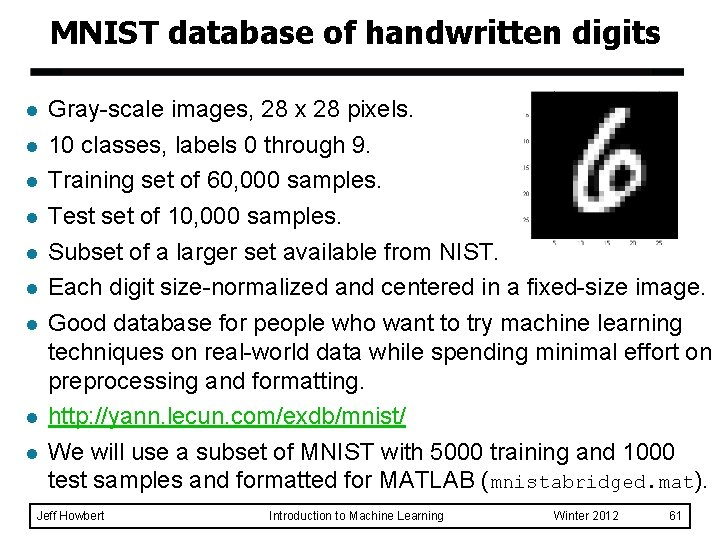MNIST database of handwritten digits l l l l l Gray-scale images, 28 x 28 pixels. 10 classes, labels 0 through 9. Training set of 60, 000 samples. Test set of 10, 000 samples. Subset of a larger set available from NIST. Each digit size-normalized and centered in a fixed-size image. Good database for people who want to try machine learning techniques on real-world data while spending minimal effort on preprocessing and formatting. http: //yann. lecun. com/exdb/mnist/ We will use a subset of MNIST with 5000 training and 1000 test samples and formatted for MATLAB (mnistabridged. mat). Jeff Howbert Introduction to Machine Learning Winter 2012 61MATLAB interlude matlab_demo_04. m Part C Jeff Howbert Introduction to Machine Learning Winter 2012 62Model validation l Every (useful) model offers choices in one or more of: – model structure u e. g. number of nodes and connections – types and numbers of parameters u e. g. coefficients, weights, etc. Furthermore, the values of most of these parameters will be modified (optimized) during the model training process. l Suppose the test data somehow influences the choice of model structure, or the optimization of parameters … l Jeff Howbert Introduction to Machine Learning Winter 2012 63Model validation The one commandment of machine learning TRAIN on TEST Jeff Howbert Introduction to Machine Learning Winter 2012 64Model validation Divide available labeled data into three sets: l Training set: – Used to drive model building and parameter optimization l Validation set – Used to gauge status of generalization error – Results can be used to guide decisions during training process typically used mostly to optimize small number of high-level meta parameters, e. g. regularization constants; number of gradient descent iterations u l Test set – Used only for final assessment of model quality, after training + validation completely finished Jeff Howbert Introduction to Machine Learning Winter 2012 65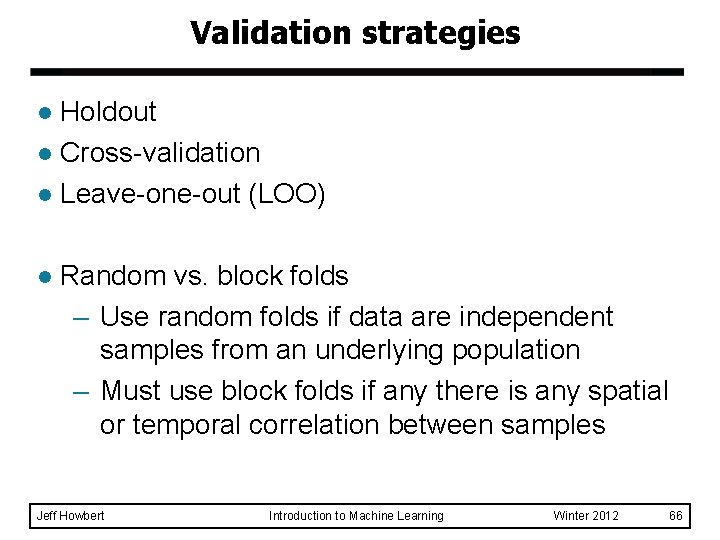Validation strategies Holdout l Cross-validation l Leave-one-out (LOO) l l Random vs. block folds – Use random folds if data are independent samples from an underlying population – Must use block folds if any there is any spatial or temporal correlation between samples Jeff Howbert Introduction to Machine Learning Winter 2012 66Validation strategies l Holdout – Pro: results in single model that can be used directly in production – Con: can be wasteful of data – Con: a single static holdout partition has the potential to be unrepresentative and statistically misleading l Cross-validation and leave-one-out (LOO) – Con: do not lead directly to a single production model – Pro: use all available data for evaulation – Pro: many partitions of data, helps average out statistical variability Jeff Howbert Introduction to Machine Learning Winter 2012 67